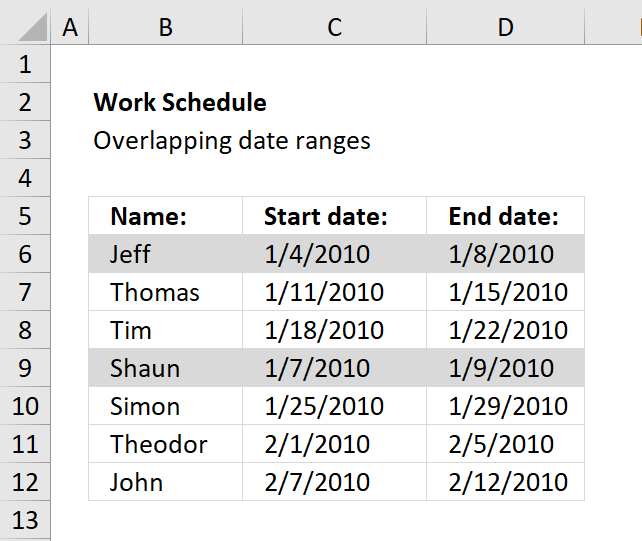Author: Oscar Cronquist Article last updated on November 14, 2018The image above demonstrates a conditional formatting formula that colors a record if there is at least one record that overlaps.

The first and fourth record are highlighted because the date ranges overlap. 1/4/2010 - 1/8/2010 overlaps with 1/7/2010 - 1/9/2010.

Here are the steps needed to create a conditional formatting formula

1. Select cell range B6:D12.
2. Press with left mouse button on "Home" tab.
3. Press with left mouse button on "Conditional Formatting" button.
4. Press with left mouse button on "New Rule..".
5. Press with left mouse button on "Use a formula to determine which cells to format".
6. Type =SUMPRODUCT((\$C6<=\$D\$6:\$D\$12)*(\$D6>=\$C\$6:\$C\$12))>1 in "Format values where this formula is TRUE" window.
7. Press with left mouse button on "Format.." button.
8. Press with left mouse button on "Fill" tab.
9. Pick a color. This color is used to highlight records that overlap.
10. Press with left mouse button on "OK".
11. Press with left mouse button on "OK".
12. Press with left mouse button on "OK".

### Explaining conditional formatting formula

=SUMPRODUCT((\$C6<=\$D\$6:\$D\$12)*(\$D6>=\$C\$6:\$C\$12))>1

Step 1 - Filter records where (\$C6<=\$D\$6:\$D\$12) is TRUE

\$C6<=\$D\$6:\$D\$12 returns an array of TRUE and/or FALSE if date in cell C6 is smaller or equal to each date in \$D\$6:\$D\$12.

All dates in\$D\$6:\$D\$12 are larger than \$C6 so the returning array is (TRUE, TRUE, TRUE,TRUE, TRUE, TRUE,TRUE)

Step 2 - Filter records where (\$D6>=\$C\$6:\$C\$12) is TRUE

(\$D6>=\$C\$6:\$C\$12) returns an array of TRUE and/or FALSE if date in cell D6 is bigger or equal to each date in \$C\$6:\$C\$12.

The returning array is (TRUE, FALSE, FALSE,TRUE, FALSE, FALSE,FALSE)

Step 3 - Putting it all together

(\$C6<=\$D\$6:\$D\$12)* (\$D6>=\$C\$6:\$C\$12)) returns the following array (1,0,0,1,0,0,0).

That means array number one and four is overlapping C6:D6) but in this case we just want to know if any date range is overlapping.

=SUMPRODUCT((\$C6<=\$D\$6:\$D\$12)*(\$D6>=\$C\$6:\$C\$12)) sums the array (1,0,0,1,0,0,0) and returns 2.

If the formula returns a number bigger than one there is at least one overlapping date range.

=SUMPRODUCT((\$C6<=\$D\$6:\$D\$12)*(\$D6>=\$C\$6:\$C\$12))>1 returns TRUE or FALSE.

Recommended articles

How to use the SUMPRODUCT function
The SUMPRODUCT function calculates the product of corresponding values and then returns the sum of each multiplication.

### Get excel *.xls file

highlight overlapping dates.xls